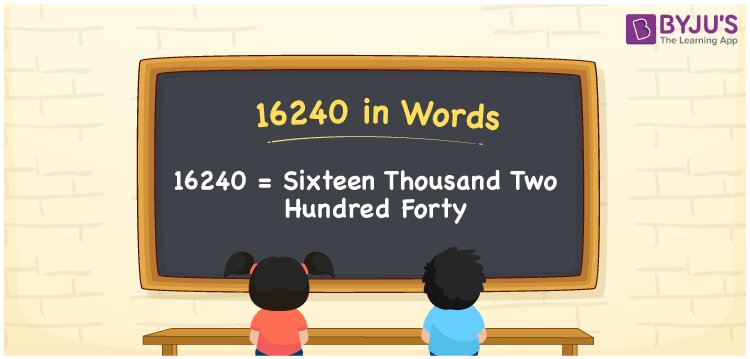# 16240 in Words

16240 in words is written as Sixteen thousand two hundred forty. In both the International System of Numerals and the Indian System of Numerals, 16240 is written as Sixteen thousand two hundred forty. The number 16240 is a Cardinal Number as it represents some quantity. For example, “the Smart-TV costs 16240 rupees”, 16240 expresses the TV cost.

 16240 in Words Sixteen thousand two hundred forty Sixteen thousand two hundred forty in number 16240

## 16240 in English Words

16240 in English words is read as “Sixteen thousand two hundred forty”.## How to Write 16240 in Words?

To write 16240 in words, we shall use the place value chart. In the place value chart, put 1 in the ten thousands, 6 in the thousands, 2 in hundreds, 4 in the tens and 0 in the ones, respectively. Let us make a place value chart to write the number 16240 in words.

 Ten Thousands Thousands Hundreds Tens Ones 1 6 2 4 0

Thus, we can write the expanded form as

1 × Ten Thousand + 6 × Thousand + 2 × Hundred + 4 × Ten + 0 × One

= 1 × 10000 + 6 × 1000 + 2 × 100 + 4 × 10 + 0 × 1

= 10000 + 6000 + 200 + 40 + 0

= 16240

= Sixteen thousand two hundred forty.

16240 is the natural number, the successor of 16239 and the predecessor of 16241.

16240 in words – Sixteen thousand two hundred forty

• Is 16240 an odd number? – No
• Is 16240 an even number? – Yes
• Is 16240 a perfect square number? – No
• Is 16240 a perfect cube number? – No
• Is 16240 a prime number? – No
• Is 16240 a composite number? – Yes

## Frequently Asked Questions on 16240 in Words

Q1

### How to write 16240 in words?

16240 in words is written as Sixteen thousand two hundred forty.
Q2

### How to write 16240 in the International and Indian System of Numerals?

In both, the system of numerals, 16240 in words, is written as Sixteen thousand two hundred forty.
Q3

### How to write 16240 in a place value chart?

In the place value chart, write 1 in the ten thousand, 6 in the thousands, 2 in hundreds, 4 in the tens and 0 in the ones, respectively.